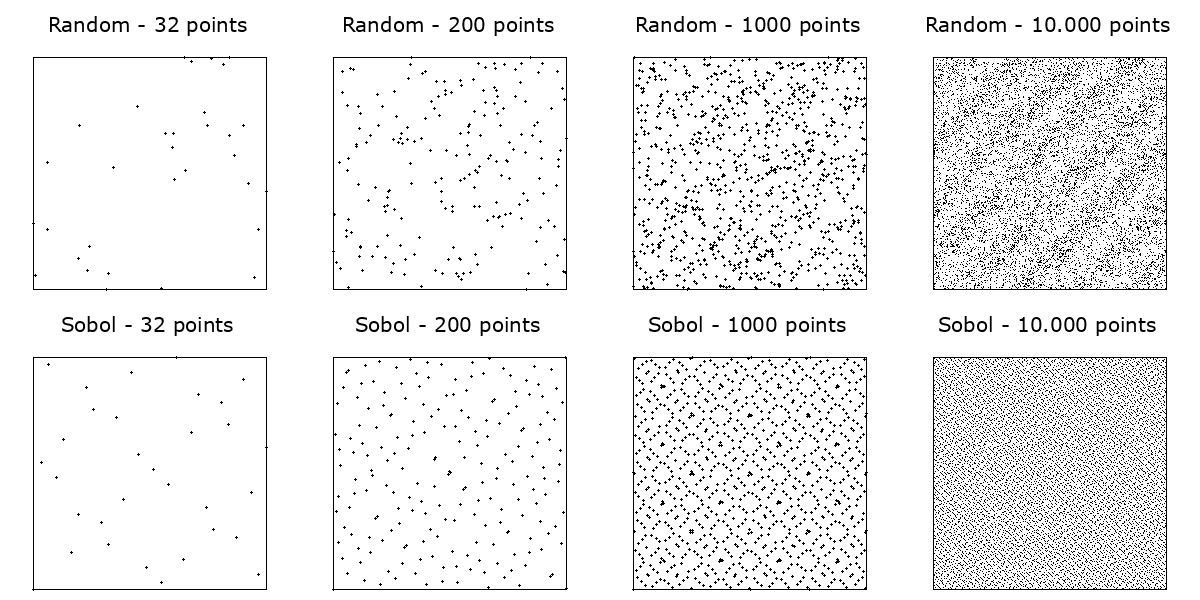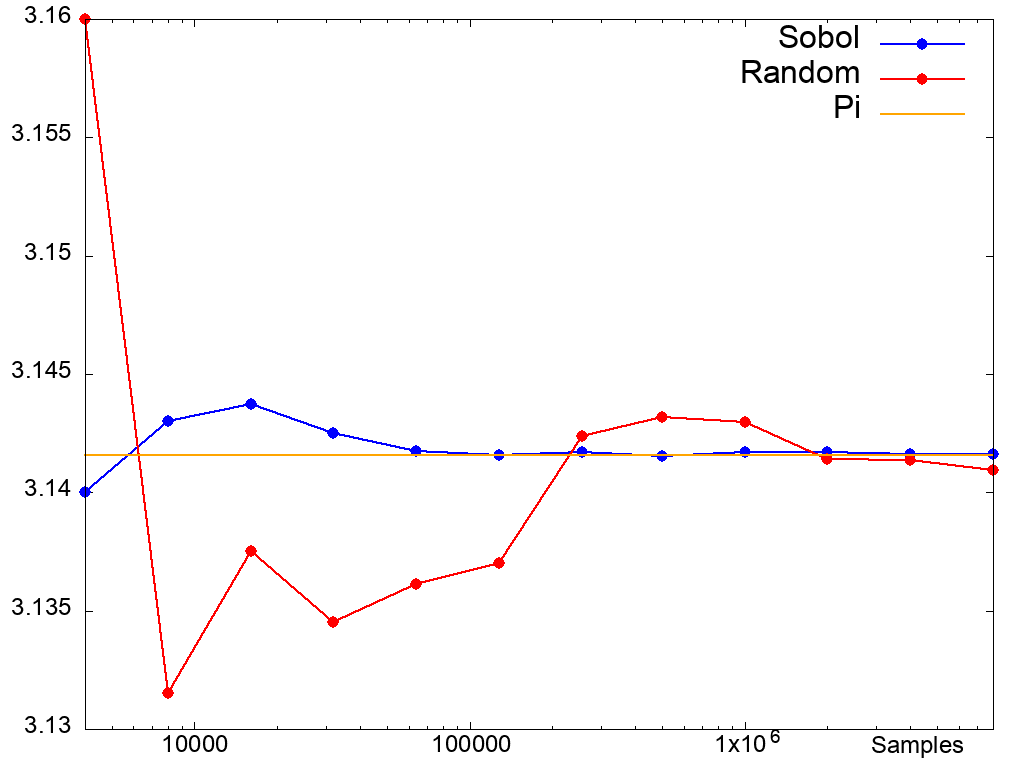Fork me on GitHub

# End of a language feature

Posted on August 15, 2022 by Troels Henriksen

I am not sure there is an established definition of the term language feature. I would probably define it as a facility that, if removed from the language, cannot be defined in terms what remains. That certainly covers syntax and type systems in most languages.

A more intrinsic definition is that a language feature is any part of the language that is implemented in the compiler itself, even if a semantically identical (but perhaps less efficient) version could be implemented in library code.

A very pragmatic definition, suited to people who have little patience with semantic games, is that a language feature is anything listed in the documentation in the chapter titled “Language Features”.

Most of Futhark’s most important programming affordances, namely the parallel array combinators such as `map` and `reduce`, look like ordinary higher-order functions and receive no special treatment from the type checker. But the compiler does treat them specially when desugaring the source program, representing them using distinct constructs in the intermediate core language, and also treating them specially in most optimisation passes. This makes them language features by the intrinsic definition above, although see this post about which of them are strictly necessary from a semantic and cost model perspective.

But to get to the point: we recently removed some of the array combinators from the language. Futhark has become fairly stable recently, and it is rare that we break programs. In this post I will justify why we saw fit to do so anyway, and why it probably does not matter. I am a master of programming language marketing, so I will start by describing in detail why the feature we just removed is actually very good and useful.

### Sequentialising parallelism

One of the basic ways to parallelise a workload is to split it into chunks, assign each chunk to a thread, have each thread compute a partial result sequentially, and then combine the per-thread results into a complete result. The same basic principle applies whether you are using a dual-core laptop from 2006 or a 2022 GPU that can sustain a hundred thousand threads in flight. Ultimately, within each thread, runs a sequential loop that does the actual work.

Consider using this approach to parallelise a reduction:

``reduce (+) 0 A``

If we have p threads available and A contains n elements, then we split A into p chunks of n/p elements each. For simplicity we’ll assume n is divisible by p. Now we need to come up with a loop for each thread. Fortunately, we can always take any parallel operation (such as `reduce`) and sequentialise it. In Futhark we can write such a chunked summation as

``unflatten p (n/p) A |> map (foldl (+) 0)``

which gives us back a small array of p partial results that can be summed sequentially.

The interesting question is how to form the sequential loop. In the example above we used a `foldl` with an operator and initial value derived from the original `reduce`. Sequentialisation of parallel code is always possible, but are there problems where it might make sense to use a different algorithm for the sequential loop? Yes! Such cases do exist.

### Sobol numbers

Suppose you forgot the value of 𝜋. The most straightforward way to recall this important constant is to draw a square on your wall, inscribe a circle within it, put on a blindfold, and throw uniformly distributed random darts at the wall. The ratio of darts that hit the circle relative to those that hit the rest of the square will asymptotically approach 𝜋/4. Such Monte Carlo methods are used in various domains to approximate unknown functions. Our original application was pricing the value of a financial option as the average value over a large set of randomly sampled future market movements.

Using traditional pseudo-random numbers, the convergence rate (the speed at which we approach the “true value”) is 1/n. Truly random numbers are not actually what we desire - when we throw the darts, we want to quickly cover the entire two-dimensional space, and excessive “clumping”, which will certainly happen with random numbers, is not productive.

Sobol numbers are quasi-random numbers that are not statistically random in the normal sense of the word, but rather try to quickly cover the space of interest. Here’s an example of how that might look visually:Two-dimensional points generated with an LCG compared to points drawn from a Sobol sequence. Note the much lower degree of clumping when using Sobol numbers.

Using Sobol numbers for Monte Carlo simulation gives us a convergence rate of 1/√n. The following graph plots the number of samples against the approximated value of 𝜋:Estimated value of 𝜋 as a function of the number of points, for pseudorandom points and quasi-random points drawn from a Sobol sequence. It is clear that using Sobol numbers converge much faster.

So how do we generate Sobol numbers? In contrast to most standard pseudorandom algorithms, which usually support only sequential generation, the independent formula tells us how to compute the ith Sobol number given some seed. The seed for Sobol number generation is a set of direction vectors that also have a length L. For the rest of this post I’ll assume they are a global variable or similar, so we won’t have to ferry them around. If we want to generate n Sobol numbers we can just `map`:

``map independent (iota n)``

Generating a single D-dimensional Sobol point from direction vectors of length L has complexity O(DL), so generating n Sobol numbers with the independent formula has complexity O(nDL).

There is also another way of generating Sobol numbers. The so-called recurrent formula can generate n Sobol numbers following some initial number x in time O(nD), making it asymptotically more efficient than the asymptotic formula. We can combine the independent and recurrent formula to compute n Sobol numbers starting from the ith one:

``recurrent (independent i) n``

This entire manoeuvre has complexity O(nD+DL). Unfortunately, parallelising the recurrent formula requires a `scan` of depth O(log(n)), so it is not as parallel as a `map` with span O(1). In practice the recurrent formula is usually best for sequential execution. Ideally each thread would use the recurrent formula, with the results of different threads concatenated to form the full sequence.

Unfortunately, the traditional data-parallel programming vocabulary is based on maximising parallelism, and letting the compiler decide when and how to exploit it on a concrete machine by synthesising sequential code from parallel specifications when needed. But the independent and recurrent formulae are very different (see this paper for the actual code), and a compiler cannot be expected to synthesise one from the other. We need a programming construct that allows the programmer to explicitly provide the “sequential loop”. In Futhark, we made this available as the function `map_stream`:

``val map_stream [n] 'a 'b : (f: (k: i64) -> [k]a -> [k]b) -> (as: [n]a) -> [n]b``

We can now generate Sobol numbers with:

``map_stream (\chunk_size chunk -> recurrent (independent chunk) chunk_size) (iota n)``

The compiler or run-time system will split the input into as many chunks as appropriate for the hardware, and use the provided function to process each chunk sequentially. Note how this formulation allows the compiler to generate fully sequential code (by setting the chunk size to n), maximally parallel code (by using n chunks of 1 element each), or anything in between.

Some properties must hold for this to make sense. Intuitively, no matter how the input array is split, we must obtain the same result. Formally, the function must be a list homomorphism under concatenation. This is not something the compiler verifies - it just assumes that the code is correct. We will return to this issue.

### Histograms

Another problem that is suitable for this approach is histograms. Given an array of n integers A and an integer k, a histogram produces an array B of size k, where B[i] is the number of times i occurs in A.

Histograms are somewhat annoying to express efficiently with standard data-parallel operations, as the result array elements depend on input array elements in ways that are not known until run-time. You can do it by sorting the input array to put identical elements next to each other and then perform an irregular segmented reduction, but this requires a lot of data movement.

However, computing a histogram sequentially is quite easy:

``````def histogram (k: i64) (n: i64) (A: [n]i64) : [k]i64 =
loop res = replicate k 0 for i < n do
res with [A[i]] = res[A[i]] + 1``````

Also, combining two histograms can be done by elementwise vector addition (`map2 (+)` in Futhark). For these cases we have the `reduce_stream` function:

``val reduce_stream [n] 'a 'b : (op: b -> b -> b) -> (f: (k: i64) -> [k]a -> b) -> (as: [n]a) -> b``

Which we can use like this:

``reduce_stream (map2 (+)) (histogram k)``

Now each thread computes a histogram for a chunk of the input, after which these partial histograms are added together. This is quite efficient as long as the histogram size k is not too large.

One interesting property is that when computing histograms, the chunks we are working on do not need to be contiguous parts of the input. Using interleaved chunks can have locality advantages on SIMD machines such as GPUs, so for this purpose Futhark we have functions `reduce_stream_per` and `map_stream_per` with the same semantics as the non-`per` versions, but where the programmer solemnly swears that it is acceptable for the chunk to not be a contiguous sub-sequence of the input array.

### Now forget all of that

Now to the actual news: Futhark no longer supports `map_stream`, `reduce_stream`, or their permuted variants. The idea behind them remains sound, but we found very few problems that could make use of them. In particular, histograms are directly supported by the compiler since 0.7.1. Efficient Sobol number generation is still useful, but in every concrete application we looked at, the work you do after Sobol generation dominates, so the actual impact was minor. (Also, improvements to our implementation of `scan` means that `recurrent` is actually faster in all cases on some GPUs.) But beyond these two examples, I don’t remember `map_stream` or `reduce_stream` seeing any use. And perhaps tellingly, I don’t remember any use by anyone who is not part of the Futhark design team itself.

But still, what is wrong with keeping a feature that is rarely - but occasionally - useful? The main cost was in compiler complexity. While chunking is the basic strategy used for code generation in all of Futhark’s parallel constructs, the streaming combinators were the only ones that exposed chunking in the IR itself. This required a lot of bespoke compiler infrastructure that served solely to support this feature. Removing the streaming combinators saved us over a thousand lines of code, much of which was likely not well tested.

Further, not only were these combinators rarely used; they were also quite difficult to use correctly. The subtle requirements regarding splitting, particularly for the permuted variants, were extremely easy to violate without noticing. Anecdotally, it is my recollection that we got it wrong every time we wrote new code with them. Combine un-checked subtle algebratic requirements with functions that make nebulous promises of being “faster” and you’re left with a device which attracts more bugs than a UV lamp in a nighttime jungle.

Unchecked algebraic requirements are not unseen in Futhark - even the humble `reduce` requires a monoid - but the combination of extremely rare use, unclear performance advantages, and a nontrivial compiler maintenance burden, was just too much. It was time to let these combinators move on to Valhalla.

This is an ex-feature.